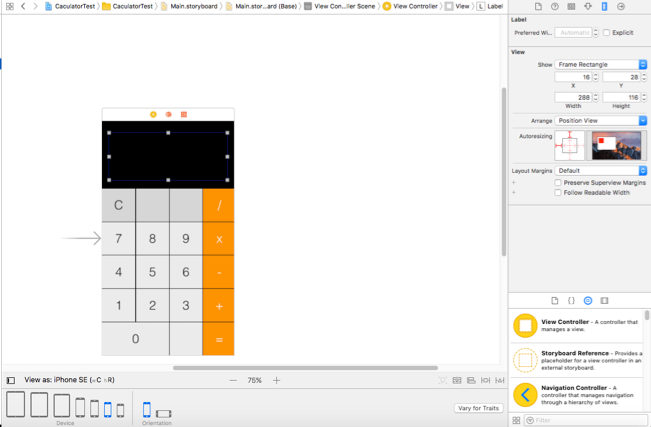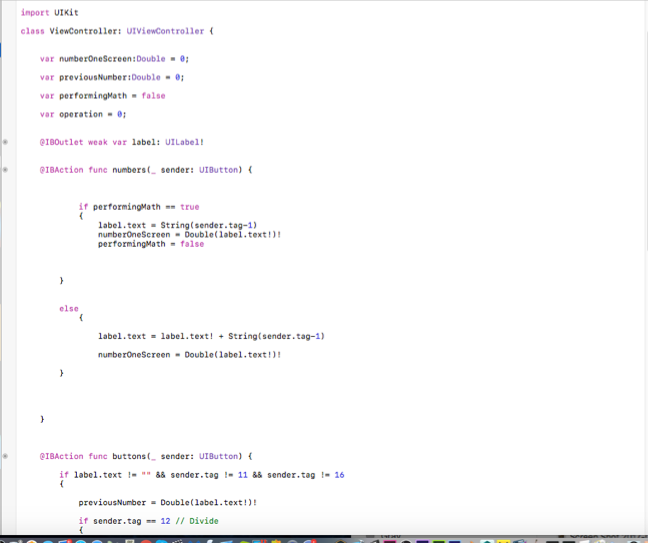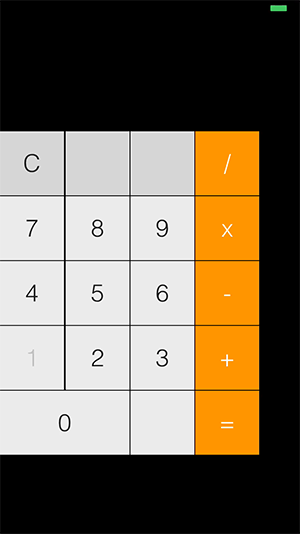## App Research: Calculator

App Research: Calculator

This week I created a simple calculator in Xcode. The calculator’s interface was designed using Xcode ’s tools. The interface contains buttons and labels as shown below in figure 1.Figure 1: Calculator’ s Interface

After the interface was designed, the operations such as addition , division and multiplication were executed using Swiftcode. In figure 2 it shows the code that was written to execute the operations.Figure 2: Script written to execute the calculator ’s operations

The end result is shown in figure 3 below.Figure 3: Calculator’ s End Result